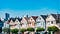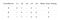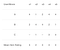Source: unsplash — Free stock images

Similarity and Distance Metrics for Data Science and Machine Learning

Applied in Recommendation Systems

In a previous article introducing Recommendation Systems, we mentioned several times the concept of ‘similarity measures’. Why? Because in Recommendation Systems, both Content-Based filtering and Collaborative filtering algorithms, use some specific similarity measure to find how equal two vectors of users or items are in between them. So in the end, a similarity measure is not more than the distance between vectors.

Note: remember that all my work, including the specific repository with the application of all this content and more about Recommendation Systems, is available in my GitHub profile.

In any kind of algorithm, the most common similarity measure is finding the cosine of the angle between vectors, i.e. cosine similarity. Suppose A is user’s A list of movies rated and B is user’s B list of movies rated, then the similarity between them can be calculated as:

Mathematically, the cosine similarity measures the cosine of the angle between two vectors projected in a multi-dimensional space. When plotted on a multi-dimensional space, the cosine similarity captures the orientation (the angle) of each vector and not the magnitude. If you want the magnitude, compute the Euclidean distance instead.

The cosine similarity is advantageous because even if the two similar documents are far apart by the Euclidean distance because of the size (like one word appearing a lot of times in a document or a user seeing a lot of times one movie) they could still have a smaller angle between them. Smaller the angle, the higher the similarity.

Take the following example from www.machinelearningplus.com:

The above image is counting the number of appearances of the word ‘sachin’, ‘dhoni’ and ‘cricket’ in the three documents shown. According to that, we could plot these three vectors to easily see the difference in between measuring the cosine and Euclidean distance for these documents:

Now, Regular Cosine Similarity by the definition reflects differences in direction, but not the location. Therefore, using the cosine similarity metric does not consider for example the difference in ratings of users. Adjusted cosine similarity offsets this drawback by subtracting respective user’s average rating from each co-rated pair, and is defined as below:

Let’s take the following example from Stackoverflow to better explain the difference between cosine and adjusted cosine similarity:

Assume a user give scores in 0~5 to two movies.

Intuitively we would say user b and c have similar tastes, and a is quite different from them. But the regular cosine similarity tells us a wrong story. IN cases like this, calculating the adjusted cosine similarity would give us a better understanding of the resemblance between users.

By the way, in our previous article about Recommendation Systems, we presented the following function to find the adjusted cosine similarity:

from scipy import spatialdef adjusted_cos_distance_matrix(size, matrix, row_column):
distances = np.zeros((size,size))
if row_column == 0:
M_u = matrix.mean(axis=1)
m_sub = matrix - M_u[:,None]
if row_column == 1:
M_u = matrix.T.mean(axis=1)
m_sub = matrix.T - M_u[:,None]
for first in range(0,size):
for sec in range(0,size):
distance = spatial.distance.cosine(m_sub[first],m_sub[sec])
distances[first,sec] = distance
return distances

And you can use this function in a very easy way, just feeding:

1. ‘matrix’: that’s just the original matrix of ratings, views or whatever you’re measuring in between users and items of your business
2. ‘row_columns’: indicating 1 if you’ll be measuring distances in between columns and 0 for distances in between rows
3. ‘size’: for the desired size of the resultant matrix. That, when finding users or items similarity it’s going to be just the number of users or items. So if you have 500 unique users, you’ll obtain a distance matrix of 500x500

Take the following example as a reference:

Finally, let’s briefly review some other methods that can be used to calculate the similarity for recommendation systems, but also for any other distance-based algorithm in Machine Learning:

• Euclidean distance: similar items will lie in close proximity to each other if plotted in n-dimensional space.
• Pearson’s correlation or correlation similarity: it tells us how much two items are correlated. The higher the correlation, the higher the similarity.
• Mean squared difference: is about finding the average squared divergence in between users ratings. MSE puts more weight into penalizing larger errors.

And then:

Where |𝐼𝑢𝑣| is just the number of items rated by both users 𝑢 and 𝑣.

Examples of user-user and item-item similarities

Let’s briefly remember how Collaborative filtering works using an example from our previous introductory article about Recommendation Systems: suppose I like the following books: ‘The blind assassin’ and ‘A Gentleman in Moscow’. And my friend Matias likes ‘The blind assassin’ and ‘A Gentleman in Moscow’ as well, but also ‘Where the crawdads sing’. It seems that Matias and I have both the same interests. So you could probably affirm I would like ‘Where the crawdads sing’ too, even though I didn’t read it. And this is exactly the logic behind collaborative filtering, with the only exception that you can compare users in between them, as well as compare items.

Let’s visualize the difference between computing use-user and item-item similarities for a recommendation system:

User-user similarity

Item-item similarity

Now, understanding this, let’ illustrate some of the measures we presented taking the following examples from our friend from Analytics Vidhya, which I found particularly clear for both, user-user and item-item similarity:

• User-user similarityImage and example are taken from Analytics Vidhya

Image and example are taken from Analytics Vidhya

Here we have a user movie rating matrix. To understand this in a more practical manner, let’s find the similarity between users (A, C) and (B, C) in the above table. Common movies rated by A and C are movies x2 and x4 and by B and C are movies x2, x4 and x5. Knowing this, let’s find the Pearson’s correlation or correlation similarity:

The correlation between user A and C is more than the correlation between B and C. Hence users A and C have more similarity and the movies liked by user A will be recommended to user C and vice versa.

• Item-item similarityImage and example are taken from Analytics Vidhya

Here the mean item rating is the average of all the ratings given to a particular item (compare it with the table we saw in user-user filtering). Instead of finding the user-user similarity, we find the item-item similarity. To do this, first we need to find such users who have rated those items and based on the ratings, the similarity between the items is calculated. Let us find the similarity between movies (x1, x4) and (x1, x5). Common users who have rated movies x1 and x4 are A and B while the users who have rated movies x1 and x5 are also A and B.

The similarity between movie x1 and x4 is more than the similarity between movie x1 and x5. So based on these similarity values, if any user searches for movie x1, they will be recommended movie x4 and vice versa.

Well, this is all for now about Recommendation Systems. However, remember that similarity measures and distance metrics appear throughout machine learning as a very fundamental concept. So I hope you’ve found this content useful not only to improve the performance of your Recommender ;)

If you enjoyed this post, don’t forget to check out some of my last articles, like 10 tips to improve your plotting skills, 6 amateur mistakes I’ve made working with train-test splits or Web scraping in 5 minutes. All of them and more available in my Medium profile.

Get in touch also by…

See in you in the next post!

Cheers.

Written by

Gonzalo Ferreiro Volpi

A network of data thought leaders, sharing lessons learned, in preparation for the future 🚀

Welcome to a place where words matter. On Medium, smart voices and original ideas take center stage - with no ads in sight. Watch
Follow all the topics you care about, and we’ll deliver the best stories for you to your homepage and inbox. Explore
Get unlimited access to the best stories on Medium — and support writers while you’re at it. Just \$5/month. Upgrade